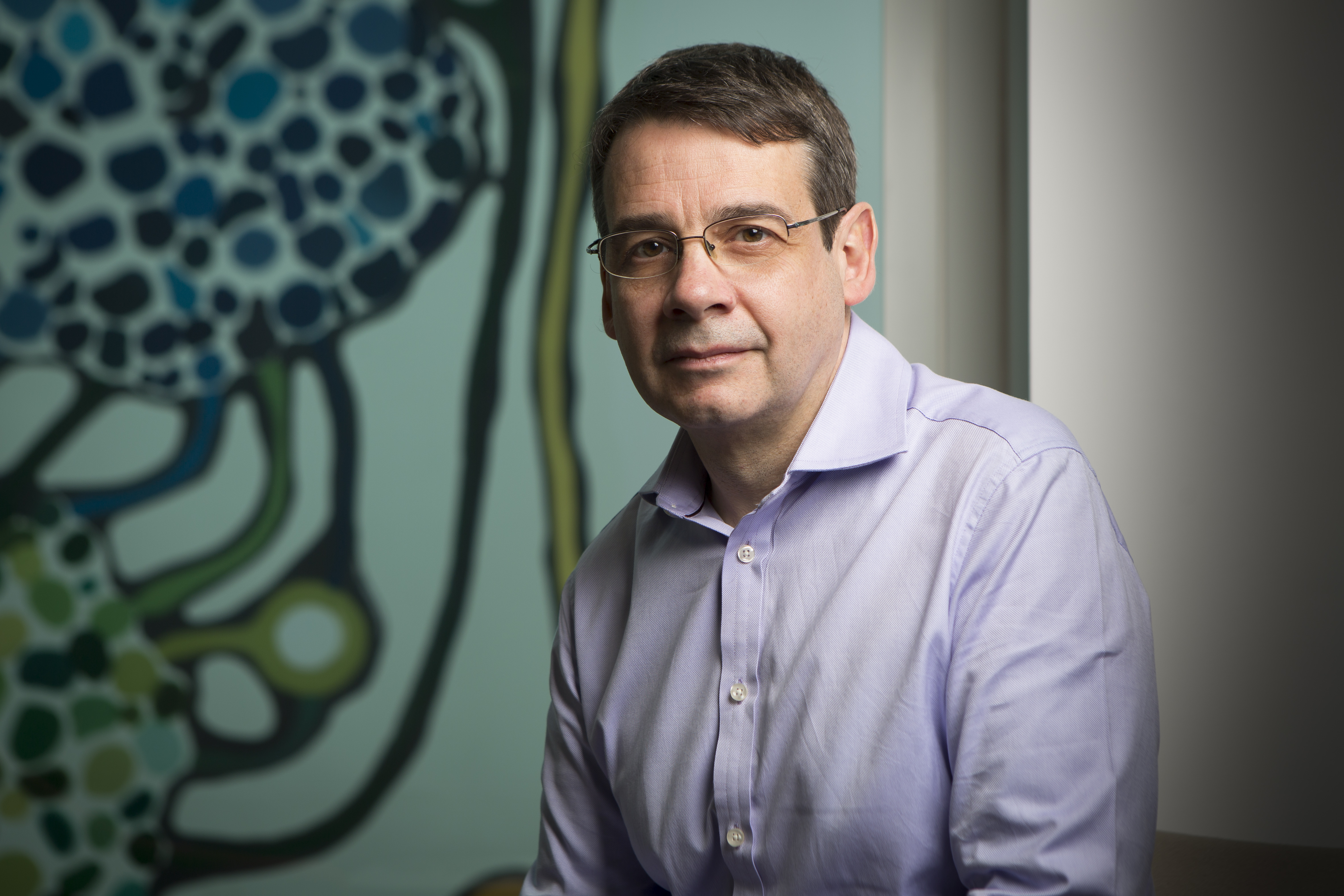# Proof Engineering, from the Four Colour to the Odd Order Theorem

Thirty-five years ago, computers made a dramatic debut in mathematics with the famous proof of the Four Colour Theorem by Appel and Haken. Their role has been expanding recently, from computational devices to tools that can tackle deduction and proofs too complex for (most) human minds, such as the Kepler conjecture or the Classification of Finite Simple Groups.

These new “machine” proofs entail fundamental changes in the practice of mathematics: a shift from craftsmanship, where each argument is a tribute to the ingenuity of the mathematician that perfected it, to a form of engineering where proofs are created more systematically. In addition to formal definitions and theorems, mathematical theories also contain clever, context-sensitive notations, usage conventions, and proof methods. To mechanize advanced mathematical results it is essential to capture these more informal elements, replacing informal and flexible usage conventions with rigorous interfaces, and exercise apprenticeship with precise algorithms. This can be difficult, requiring an array of techniques closer to software engineering than formal logic, but it is essential to obtaining formal proofs of graduate-level mathematics, and can give new insight as well.

In this talk, we will give several examples of such empirical formal mathematics that we have encountered in the process of mechanizing a large corpus of Combinatorics and Algebra required by the proofs of the Four Colour and Odd Order Theorem.

##### Speaker Details

Georges Gonthier is a Researcher at MSR Cambridge, which he joined in 2003, after 13 year in France at Inria (Institut National de Recherches en Informatique et Automatique) and École Polytechnique, and two years at AT&T Bell Labs. Dr. Gonthier received his Doctorat in 1988 from Université Paris XI for work on the design, semantics and implemetation of the Esterel reactive programming language. His work includes an analysis of techniques for the optimal computation of functional programs, the design and formal verification of a concurrent memory management algorithm (used since in the Ocaml and IBM Java runtimes), the join calculus model of concurrency (used in the design of the Cw language), concurrency analysis of the Ariane 5 flight software, pioneering the use of full abstraction in the analysis of security properties, a fully computer-checked proof of the famous Four Colour Theorem.

Date:
Speakers:
Georges Gonthier
•### Georges Gonthier

Principal Researcher